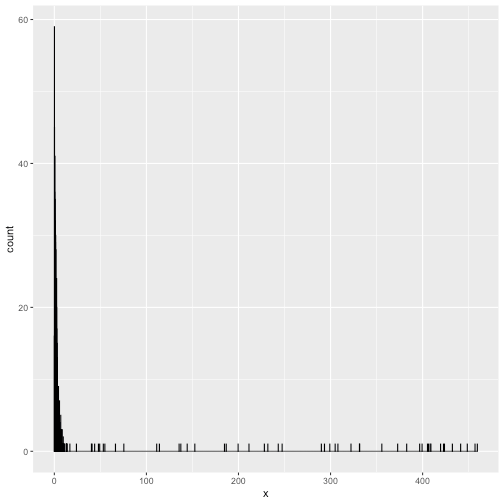Want to share your content on R-bloggers? click here if you have a blog, or here if you don't.

I guess we all use it, the good old histogram. One of the first things we are taught in Introduction to Statistics and routinely applied whenever coming across a new continuous variable. However, it easily gets messed up by outliers. Putting most of the data into a single bin or a few bins, and scattering the outliers barely visible over the x-axis. This distribution might look familiar

```library(tidyverse)
set.seed(42)
hist_data <- data_frame(x = c(rexp(1000, .5),
runif(50, 0, 500)))

ggplot(hist_data, aes(x)) +
geom_histogram(binwidth = .1, col = "black", fill = "cornflowerblue")
```Two strategies that make the above into something more interpretable are taking the logarithm of the variable, or omitting the outliers. Both do not show the original distribution, however. Another way to go, is to create one bin for all the outlier values. This way we would see the original distribution where the density is the highest, while at the same time getting a feel for the number of outliers. A quick and dirty implementation of this would be

```hist_data %>%
mutate(x_new = ifelse(x > 10, 10, x)) %>%
ggplot(aes(x_new)) +
geom_histogram(binwidth = .1, col = "black", fill = "cornflowerblue")
```Not bad. However, it now suggests incorrectly that many observations are exactly 10. I routinely make these plots for my own information, but they cannot be shared without explaining what happened to the outliers and what there original range was. Since a plot with a manual is not that great either, I recently did a hacking session into the `ggplot` object. The resulting `gg_outlier_bin` function not only indicates the range of the last bin, it also allows for a different fill color of the bin. Now we are clearly distinguishing the outlier aggregation

```gg_outlier_bin(hist_data,
"x",
cut_off_floor = NA,
cut_off_ceiling = 10,
binwidth = 0.1)
```It is still a bit experimental, but it seems to work in most situations. Below you find the function code for making histograms with outlier bins. You can also get it by installing the package accompanying this blog `devtools::install_github("edwinth/thatssorandom")`. By the way, it works on both floor and ceiling outliers. Like in the following

```data_frame(x = c(runif(100, 0, 100), rnorm(1000, 50, 2))) %>%
gg_outlier_bin("x", 45, 55, binwidth = .1)
``````gg_outlier_bin <- function(x,
var_name,
cut_off_floor,
cut_off_ceiling,
col = "black",
fill = "cornflowerblue",
fill_outlier_bins = "forestgreen",
binwidth = NULL) {

printing_min_max <- x %>% summarise_(sprintf("round(min(%s, na.rm = TRUE), 1)", var_name),
sprintf("round(max(%s, na.rm = TRUE), 1)", var_name))

ceiling_filter <- ifelse(!is.na(cut_off_ceiling),
sprintf("%s < %f", var_name, cut_off_ceiling),
"1 == 1")
floor_filter   <- ifelse(!is.na(cut_off_floor),
sprintf("%s > %f", var_name, cut_off_floor),
"1 == 1")

x_regular <- x %>% filter_(ceiling_filter, floor_filter) %>%
select_(var_name)

x_to_roll_ceiling <- x %>% filter_(
sprintf("%s >= %f", var_name, cut_off_ceiling)) %>% select_(var_name)
if (!is.na(cut_off_ceiling)) x_to_roll_ceiling[, 1] <- cut_off_ceiling

x_to_roll_floor <- x %>% filter_(
sprintf("%s <= %f", var_name, cut_off_floor)) %>% select_(var_name)
if (!is.na(cut_off_floor)) x_to_roll_floor[, 1] <- cut_off_floor

plot_obj <- ggplot(x_regular, aes_string(var_name)) +
geom_histogram(col = col, fill = fill, binwidth = binwidth)

if (!is.na(cut_off_ceiling)) {
ticks_for_ceiling <- update_tickmarks_ceiling(plot_obj, cut_off_ceiling,
printing_min_max[1,2])
plot_obj <- plot_obj +
geom_histogram(data = x_to_roll_ceiling, fill = fill_outlier_bins, col = col,
binwidth = binwidth) +
scale_x_continuous(breaks = ticks_for_ceiling\$tick_positions,
labels = ticks_for_ceiling\$tick_labels)
}

if (!is.na(cut_off_floor)) {
ticks_for_floor <- update_tickmarks_floor(plot_obj, cut_off_floor,
printing_min_max[1,1])
plot_obj <- plot_obj +
geom_histogram(data = x_to_roll_floor, fill = fill_outlier_bins,
col = col, binwidth = binwidth) +
scale_x_continuous(breaks = ticks_for_floor\$tick_positions,
labels = ticks_for_floor\$tick_labels)
}

return(plot_obj)
}

update_tickmarks_ceiling <- function(gg_obj,
co,
max_print) {
ranges <- suppressMessages(
ggplot_build(gg_obj)\$layout\$panel_ranges[])
label_to_add <- sprintf("(%s , %s)", round(co, 1), max_print)
tick_positions <- ranges\$x.major_source
tick_labels    <- ranges\$x.labels
if (overlap_ceiling(tick_positions, co)) {
tick_positions <- tick_positions[-length(tick_positions)]
tick_labels    <- tick_labels[-length(tick_labels)]
}
return(list(tick_positions = c(tick_positions, co),
}

overlap_ceiling <- function(positions, cut_off) {
n <- length(positions)
ticks_dif <- positions[n] - positions[n-1]
(cut_off - positions[n]) / ticks_dif < 0.25
}

update_tickmarks_floor <- function(gg_obj,
co,
min_print) {
ranges <- suppressMessages(
ggplot_build(gg_obj)\$layout\$panel_ranges[])
label_to_add <- sprintf("(%s , %s)", min_print, round(co, 1))
tick_positions <- ranges\$x.major_source
tick_labels    <- ranges\$x.labels
if (overlap_floor(tick_positions, co)) {
tick_positions <- tick_positions[-1]
tick_labels    <- tick_labels[-1]
}
return(list(tick_positions = c(co, tick_positions),
}

overlap_floor <- function(positions, cut_off) {
ticks_dif <- positions - positions
(positions - cut_off) / ticks_dif < 0.25
}
```